# Tangents: Definition & Properties

Instructor: Yuanxin (Amy) Yang Alcocer

Amy has a master's degree in secondary education and has taught math at a public charter high school.

In this lesson, we will cover the tangent as it is used in calculus. You will learn the process of finding the tangents of curves and will also find out what defines tangents in calculus.

## Definition

In calculus, a tangent is the line of the slope of the curve at a particular point. It is the line that touches the curve at any particular point that goes in the same direction as the curve at that point. In other words, if the curve stopped following the function at that point and went straight, that would be the tangent line.

This is what a tangent line looks like when drawn on a graph of a curve.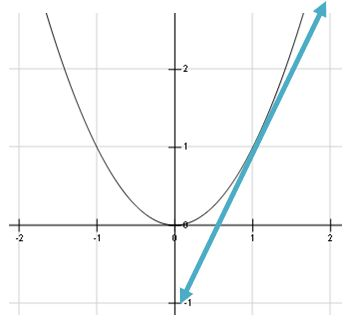The tangent just touches the curve at a point and gives the slope of the curve at that point.

## Properties

Tangents have several properties of interest. We have just touched on these in the definition section.

1. Tangents approximate the curve at a point. They give the best approximation at that point by showing you the slope of the curve at that point.

2. The tangent barely touches the curve at a point.

3. For a point on a curve to have a tangent, it must be differentiable at that point. If it is not differentiable at that point, it has no defined slope and therefore has no defined tangent since the tangent is given by the slope at that point.

## The Formula

Because the tangent is the line of the slope of a curve at any given point, the formula for the tangent involves the derivative of the curve. The derivative of the curve gives you the change of the curve at any given point which is the slope. So, evaluating the derivative at a given point will give you the slope which you can use to find the tangent line.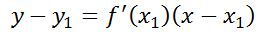The tangent line is found by using the point-slope form of a line and plugging in a given point along with the derivative evaluated at that point. The equation is then solved for y to find the equation.

## Calculating the Tangent

Let's now see how the formula works for a function. We will work on the following function to see how we can find our tangent line.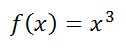We want to evaluate it at the point where x=1. To find out the exact point, we can plug in our x to find our y. Our expression f(x) is the same as y. We can interchange them at will.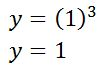Our y is 1, so our point is (1, 1).

According to our formula, have our point and now we need our derivative. We will take the derivative of our function now and follow any derivative properties that we know of.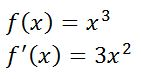We have used the power rule of derivatives to find our derivative. Now we need to evaluate our derivative at the point where x=1. We can do that by plugging in our x into the derivative to find our slope.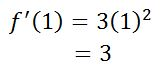Our slope is 3. Now we can plug in all our values into the point-slope form of the equation of a line and solve for y to get our tangent line equation.

To unlock this lesson you must be a Study.com Member.
Create your account

### Register to view this lesson

Are you a student or a teacher?

### Unlock Your Education

#### See for yourself why 30 million people use Study.com

##### Become a Study.com member and start learning now.
Back
What teachers are saying about Study.com
Create an account to start this course today
Used by over 30 million students worldwide